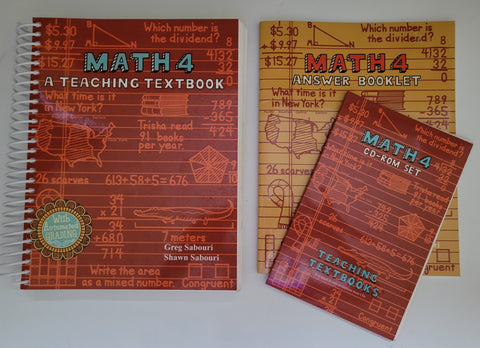# MATH 4 Teaching Textbook Set

• \$ 48.00

The Math 4 Teaching Textbook includes a 669-page consumable workbook, answer booklet and CD-Roms
Like New!

The Math 4 Teaching Textbook includes a 669-page consumable workbook (because this textbook is rented, answers will need to be written on a separate sheet of paper), a print answer booklet, and 4 CDs that contain step-by-step audiovisual solutions to every one of the thousands of homework and test problems. Topics covered include operations with whole numbers, fractions, and decimals, simple geometry concepts, units of measure, and percents. The Math 4 also has a digital gradebook that grades answers as soon as they are entered and calculates percentages for each assignment.